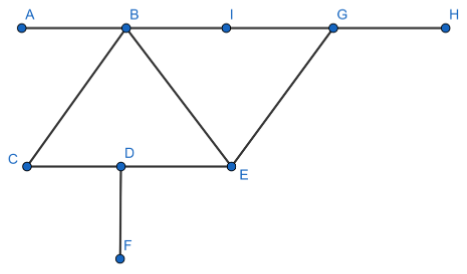Filters
Latest Questions
CBSE
Mathematics
Line segment
There are Four Points $A,B,C,D$ on a straight line the distance between $A$ and $B$ is $3cm$. $C$ and $D$ are both twice as far from $A$ as from $B$ then the distance between $C$ and $D$ is
$A)1cm$
$B)2cm$
$C)3cm$
$D)4cm$
CBSE
Mathematics
Line segment
Give a definition for line segment

CBSE
Mathematics
Line segment
How many line segments are there in the given figure?CBSE
Mathematics
Line segment
Find the ratio in which the point $(11,15)$divides the line segment joining the points $(15,5)$and $(9,20)$

CBSE
Mathematics
Line segment
Identify the symbol of line segments from the following options
\begin{align} & \left[ a \right]\overleftrightarrow{AB} \\ & \left[ b \right]\overline{AB} \\ & \left[ c \right]\overrightarrow{AB} \\ & \left[ d \right]\text{ None of these} \\ \end{align}

CBSE
Mathematics
Line segment
Tell the following statement is true (T) or false (F):
Every segment is a ray
CBSE
Mathematics
Line segment
Number of end points a line segment has
$\left( a \right)$ Three
$\left( b \right)$ None
$\left( c \right)$ Two
$\left( d \right)$ One

CBSE
Mathematics
Line segment
Let A (4, 2), B (6, 5) and C (1, 4) be the vertices of $\Delta ABC$.

(i). The median from A meets BC at D. Find the coordinates of the point D.
(ii). Find the coordinates of the point P on AD such that AP: PD = 2: 1
(iii). Find the coordinates of points Q and R on medians BE and CF respectively such that BQ: QE =2:1
(iv). What do you observe? [Note: The point which is common to all the three medians is called the centroid and this point divides each median in the ratio 2:1]
(v). If A $({x_1},{y_1})$, B $({x_2},{y_2})$ , and C $({x_3},{y_3})$ are the vertices of $\Delta ABC$, find the coordinates of the centroid of the triangle.
CBSE
Mathematics
Line segment
Prove that every line segment has one and only one midpoint.
CBSE
Mathematics
Line segment
Find the ratio in which the line segment joining the points A (3, -3) and B (-2, 7) is divided by the x-axis. Two times the x-coordinate of the point of division is
CBSE
Mathematics
Line segment
Line segment has …………………. end points.
$(a){\text{ no}} \\ (b){\text{ 2}} \\ (c){\text{ 1}} \\ (d){\text{ 3}} \\$
CBSE
Mathematics
Line segment
In what ratio is the line segment joining the points (-2, -3) and (3, 7) divided by y - axis?
(a) 5 : 1
(b) 1 : 5
(c) 2 : 3
(d) 3 : 2
Prev
1
2